# How The Golden Rule Helps You Design Top Notch Graphics

If you liked this, Share it now

I just came across an interesting article about graphic designs and maths. I am currently designing the cover for my future book; so I was really intrigued by what I’ve found. Thrilling!

If you are designing your own graphics, maybe for social media or blog purpose, look closely to these principles.

Want to be on the same creative level as Leonardo Da Vinci, Salvador Dali and the designers of the Parthenon? They all have one simple concept in common.

The Ancient Greeks were one of the first to discover a way to harness the beautiful asymmetry found in plants, animals, insects and other natural structures. They expressed this mathematical phenomenon with the Greek letter phi, but today, we call it the golden ratio—also known as the divine proportion, the golden mean, and the golden section.

What does an Italian mathematician from the 12th century have to do with marketing and design?

His mathematical concept can be applied to your graphic designs to make them more visually appealing to the viewer.

Leonardo Fibonacci discovered a special sequence of numbers called the Fibonacci sequence. It looks like this:

0, 1, 1, 2, 3, 5, 8, 13, 21, 34, 55, 89, 144 …

Notice the pattern in these numbers? Each is the sum of the previous two numbers. For instance, the next number in the sequence is 233 (89 +144).

The Fibonacci sequence creates two interesting ratios. First, divide a number in the sequence by its previous number, and you’ll get a ratio of about 1.618. For example, 34/21 = 1.619.

This ratio, 1.618, is known as the golden rule or the golden ratio

Leonardo of Pisa, or Leonardo Pisano, was the Middle Ages mathematician who discovered recurring patterns in nature. He was born somewhere around 1175 in Pisa, Italy. Leonardo grew up in North Africa, where his father, Guglielmo Bonacci, was a customs agent. The name Fibonacci (by which this math teacher is known to us today) means “filius Bonacci,” or “fi-Bonacci,” or son of Bonacci. Thus, Leonardo Pisano Fibonacci, or Leonardo Fibonacci. Mr. Bonacci’s tour of duty in North Africa introduced son Leonardo to what came to be known as “Arabic numbers,” and it was Leonardo who introduced this form of mathematics to Europe.

In 1202, young Leonardo published a landmark math textbook called Liber Abaci, in which he used the example of rabbits to show how rapidly a pair of rabbits can populate an area with their offspring. He posed the textbook question this way: “If a pair of rabbits is placed in an enclosed area, how many rabbits will be born there if we assume that every month a pair of rabbits produces another pair, and that rabbits begin to bear young two months after their birth?”

. You can observe this ratio in objects like a nautilus shell, in the way trees branch out and grow, the spirals of galaxies, and in lots of art and architecture. Another Leonardo, in this case da Vinci, used the ratio 1.618 to create the Vitruvian Man.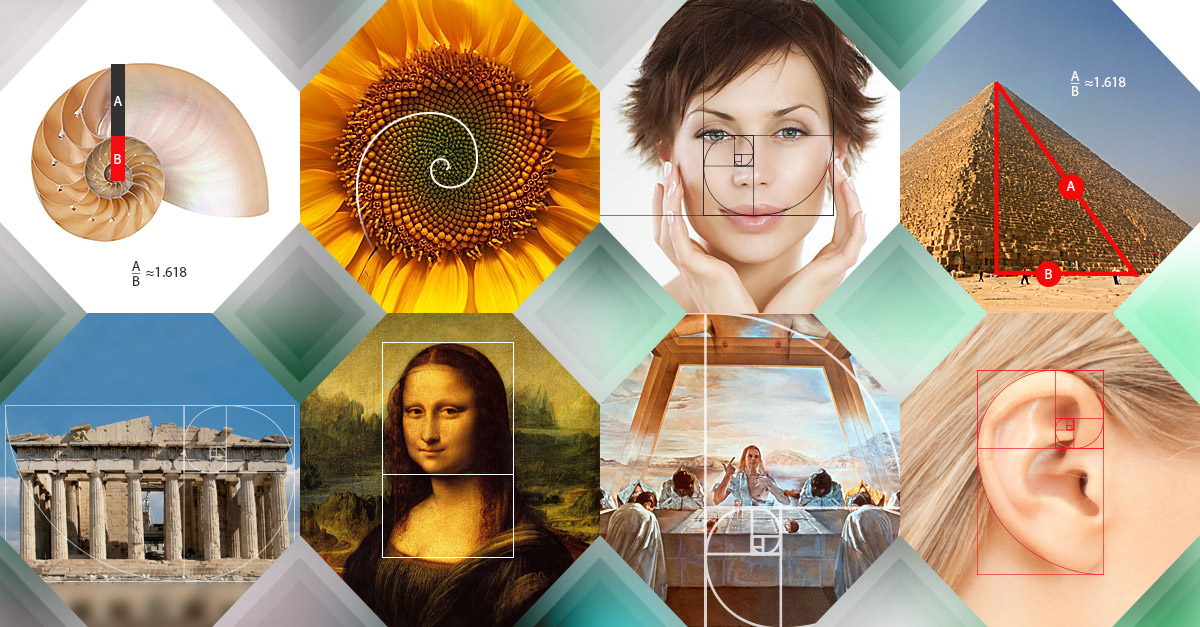##### Creating a golden rectangle

So now that we understand the basic numbers at play, here’s a more advanced technique for using those numbers in a more visual way. A “golden rectangle” is one that fits the parameters of the golden ratio—but the more times you divide a golden rectangle according to the golden ratio, the more useful it becomes.

Let’s start by creating a rectangle with golden proportions. To make this simple, we’ll start with a width of 1000 pixels and divide it by 1.618 to get a height of about 618 pixels.Now add a 618 x 618 square on the right side of the canvas, leaving behind a 382 x 618 rectangle on the left side—another golden rectangle!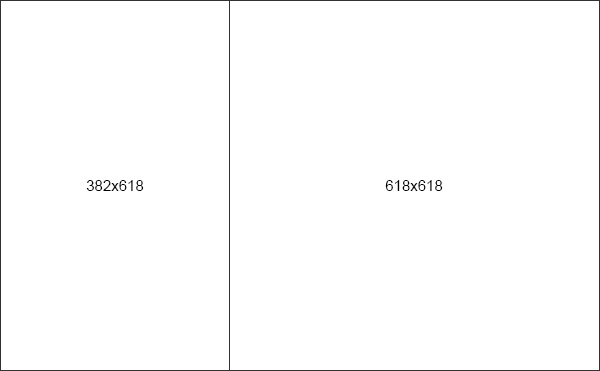If you take that new rectangle and create another square within it, you’ll end up with another golden rectangle in the leftover space, which you can then divide up again, and so on and so forth.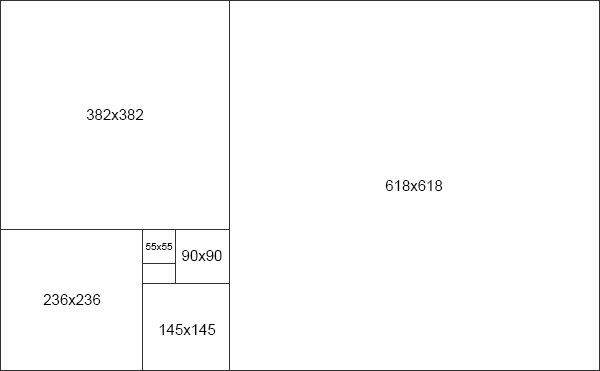Notice how each time you divide your golden rectangle, the largest dividing line kind of spirals in onto itself? That’s no accident—it forms the shape of a “golden spiral,” one of the more ubiquitous shapes that you’ll deal with when working with the golden ratio.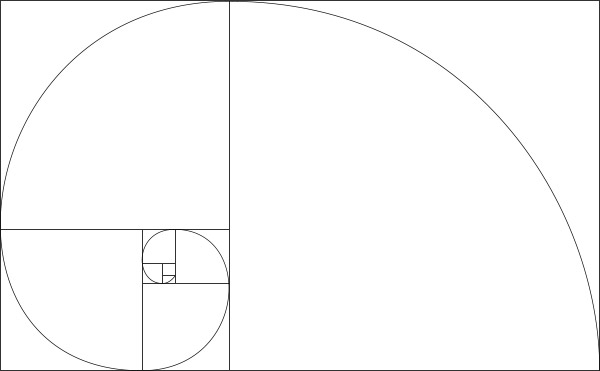## Infographic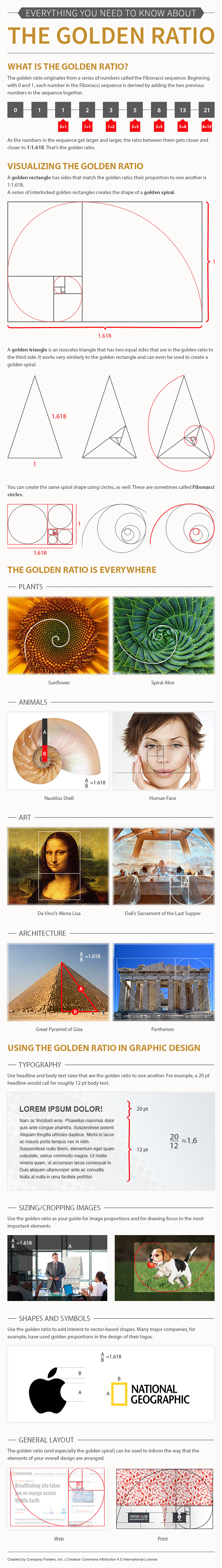Use the Golden Ratio to Create Gorgeous Designs

____________________________________

Did This Help You? If so, I would greatly appreciate it if you commented below and shared with the worldChristiane Agricola

If you liked this, Share it now